Why Binary?
The number system that you are familiar with, that you use every day, is the decimal number system, also
commonly referred to as the base-10 system. When you perform computations such as 3 + 2 = 5, or 21 –
7 = 14, you are using the decimal number system.

This system, which you likely learned in first or second grade, is ingrained into your subconscious; it’s the natural way that you think about numbers. Of course it is not just you: It is the way that everyone thinks—and has always thought—about numbers and arithmetic. Evidence exists that Egyptians were using a decimal number system five thousand years ago.

The Roman numeral system, predominant for hundreds of years, was also a decimal number system (though organized differently from the Arabic base-10 number system that we are most familiar with). Indeed, base-10 systems, in one form or another, have been the most widely used number systems ever since civilization started counting

In dealing with the inner workings of a computer, though, you are going to have to learn to think in a different number system, the binary number system, also referred to as the base-2 system.

Consider: Computers are built from transistors, and an individual transistor can only be ON or OFF (two options). Similarly, data storage devices can be optical or magnetic. Optical storage devices store data in a specific location by controlling whether light is reflected off that location or is not reflected off that location (two options).

Likewise, magnetic storage devices store data in a specific location by magnetizing the particles in that location with a specific orientation. We can have the north magnetic pole pointing in one direction, or the opposite direction (two options).

Computers can most readily use two symbols, and therefore a base-2 system, or binary number system, is most appropriate. The base-10 number system has 10 distinct symbols: 0, 1, 2, 3, 4, 5, 6, 7, 8 and 9.

The base-2 system has exactly two symbols: 0 and 1. The base-10 symbols are termed digits. The base-2 symbols are termed binary digits, or bits for short. All base-10 numbers are built as strings of digits (such as 6349).

All binary numbers are built as strings of bits (such as 1101). Just as we would say that the decimal number 12890 has five digits, we would say that the binary number 11001 is a five-bit number.

•  All computers use the binary system :
•      1.Binary number system: Base = 2.Thus there are 2 numbers: 0 and 1.
2.single binary number is called a Binary digIT, or bit.
•  Computers perform operations on binary number groups called words.
•  Today, most computers use 32- or 64-bit words:
1.Words are subdivided into 8-bit groups called bytes.
2. One-half a byte is sometimes referred to as a nibble (a term not often used anymore).

The Binary Number System
Since binary numbers can only contain the two symbols 0 and 1, numbers such as 25 and 1114000 cannot be binary numbers.

A string of eight bits (such as 11000110) is termed a byte. A collection of four bits (such as 1011) is smaller than a byte, and is hence termed a nibble

Converting Between Binary Numbers and Decimal Numbers

Converting a Binary Number to a Decimal Number

To convert a binary number to a decimal number, we simply write the binary number as a sum of powers of 2.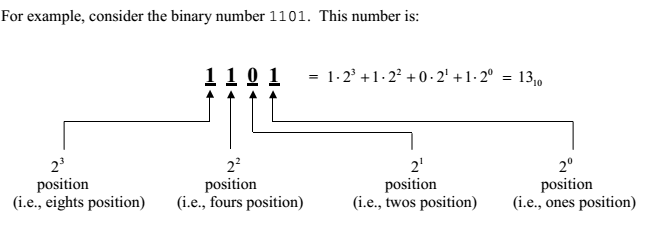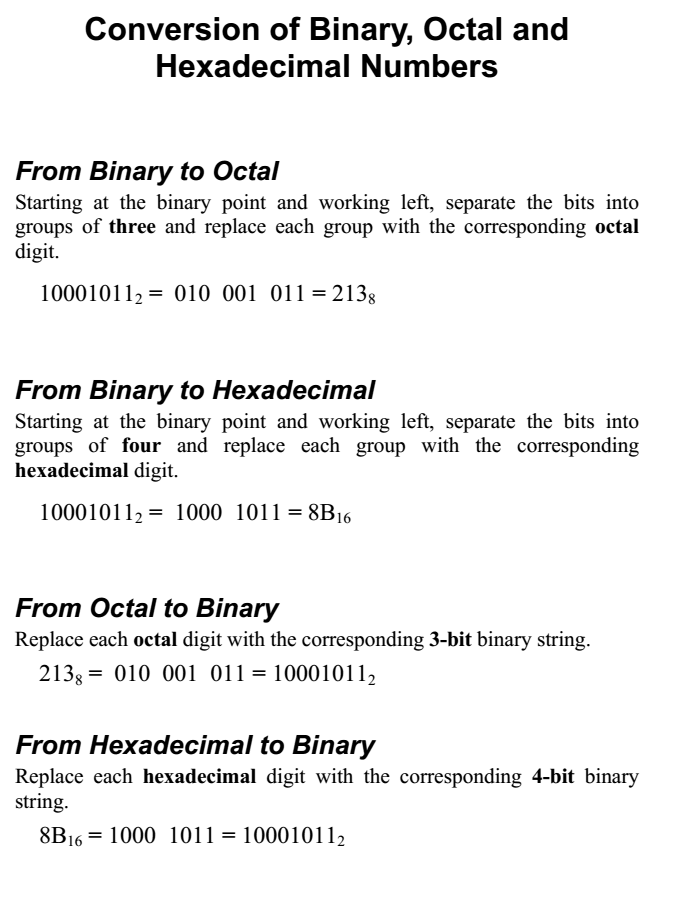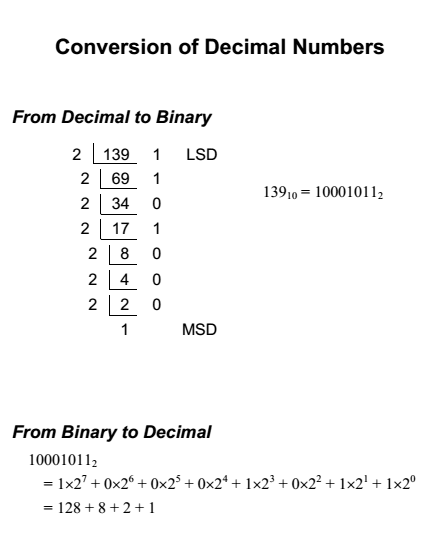Binary Addition and SubstractionThe Hexadecimal Number System

The base-16 hexadecimal number system has 16 digits (0, 1, 2, 3, 4, 5, 6, 7, 8, 9, A, B, C, D, E and F). Note that the single hexadecimal symbol A is equivalent to the decimal number 10, the single symbol B is equivalent to the decimal number 11, and so forth, with the symbol F being equivalent to the decimal number 15. Just as with decimal notation or binary notation, we again write a number as a string of symbols, but now each symbol is one of the 16 possible hexadecimal digits (0 through F).

To interpret a hexadecimal number, we multiply each digit by the power of 16 associated with that digit’s position.

For example, consider the hexadecimal number 1A9B. Indicating the values associated with the positions of the symbols, this number is illustrated as: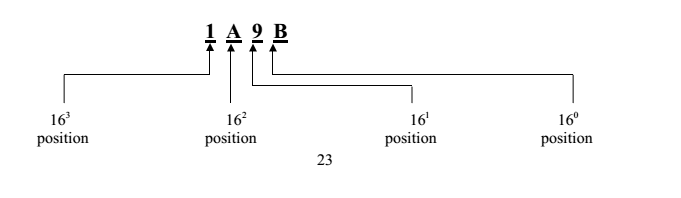Converting a Hexadecimal Number to a Decimal Number

To convert a hexadecimal number to a decimal number, write the hexadecimal number as a sum of powers of 16. For example, considering the hexadecimal number 1A9B above, we convert this to decimal as: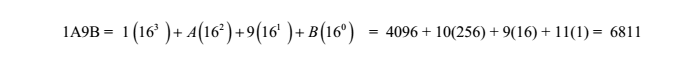Converting a Decimal Number to a Hexadecimal Number

The easiest way to convert from decimal to hexadecimal is to use the same division algorithm that you used to convert from decimal to binary, but repeatedly dividing by 16 instead of by 2. As before, we keep track of the remainders, and the sequence of remainders

forms the hexadecimal representation. For example, to convert the decimal number 746 to hexadecimal, we proceed as follows:So, the decimal number 746 = 2EA in hexadecimal

Sign-Magnitude

0 = positive

1 = negative

n bit range = -(2n-1-1) to +(2n-1-1)

4 bits range = -7 to +7

2 possible representation of zero.

2’s Complement

flip bits and add one

Sign Extend

add 0 for positive numbers

add 1 for negative numbers

You may be interested in:
Digital Electronics MCQs